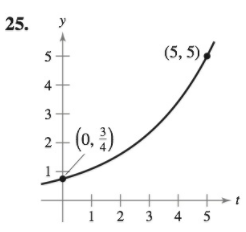Chapter 6, Problem 25RE

Chapter
Section
Textbook Problem

Finding an Exponential Function In Exercises 25–28, find the exponential function y = Cekt that passes through the two given points.To determine

To calculate: An exponential function y=Cekt that passes through two given points.

Explanation

Given:

Exponential function y=Cekt

Formula used:

lnex=x

Calculation:

Exponential function y=Cekt...... (1)

Now we put the value of t1 and y1 in equation (1)

34=Ce0C=34=0

Still sussing out bartleby?

Check out a sample textbook solution.

See a sample solution

The Solution to Your Study Problems

Bartleby provides explanations to thousands of textbook problems written by our experts, many with advanced degrees!

Get Started

Find more solutions based on key concepts